Courses

Test: Line And Angles

15 Questions MCQ Test Quantitative Aptitude for GMAT | Test: Line And Angles

Description
Attempt Test: Line And Angles | 15 questions in 30 minutes | Mock test for GMAT preparation | Free important questions MCQ to study Quantitative Aptitude for GMAT for GMAT Exam | Download free PDF with solutions
QUESTION: 1

Solution:
QUESTION: 2

If two complementary angles are in the ratio 13:5, then the angles are ________.

Solution:

Given that the ratio of two complementary angles is 13 : 5.
Let the angles are13x and 5x
We know, 13x + 5x = 90o
⇒ x = 5
Therefore, the angles are 65o and 25o.

QUESTION: 3

In the given figure, BC and EF are line segments intersecting at F. FD and AF are the angle bisectors of ∠EFC and ∠EFB respectively. Find the value ∠AFE + ∠DFE.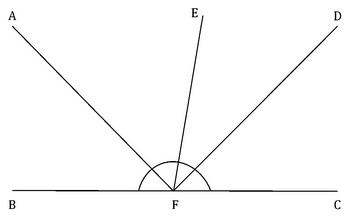Solution:
QUESTION: 4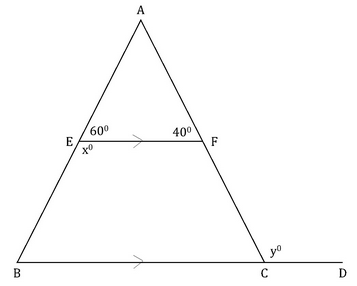In the given diagram, line segment EF and BC are parallel to each other. Find the value of (x+y).

Solution:
QUESTION: 5Three parallel lines l1, l2 and lare intersected by two parallel transversals m1 and m2. What is the measure of angle y?

Solution:

Step 1: Question statement and Inferences

We are given that three parallel lines l1, l2 and l3 are intersected by two parallel transversals m1 and m2. Also, two angles 3x-100 and 3x+10 are formed as shown in the figure.

We have to find the value of angle y.

We know that a line which intersects two or more parallel lines is called a Transversal line.

Let us name the intersecting points and understand angles associated them.To find the value of angle y, we must build a relationship between ∠B,?E,and ∠F

with the use of properties discussed above.

Step 2: Finding required values

Two parallel lines l1,&l2

are intersected by transversal m1, so

∠F=y=∠E=3x+10

(Since F and E are corresponding angles.)

So, if we get the value of x we can get the value of y.

To get the value of x, we must make a relationship between ∠Band?E.

We find that

∠B=∠D(Correspondingangles)

∠D=∠FED(Correspondingangles) and ∠FED=180−∠E (Pair of linear angles) Thus, we can say: ∠D = ∠FED = 180o−∠E

∠B=180o−∠E

Step 3: Calculating the final answer

Now, ∠B=180o−∠E

3x−100o=180o−(3x+10)

3x−100o=180o−3x−10o 6x=270o

x=45

or, yo=∠E=3×45o+10o=145o

QUESTION: 6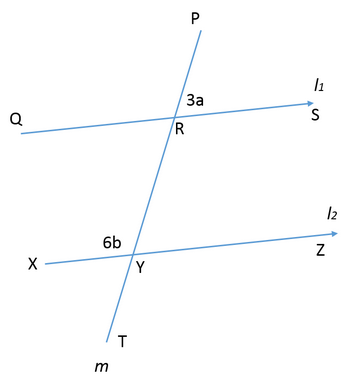If the lines l1 and l2 are parallel to each other, and the transversal line m intersects it such that ∠PRS= 3a and ∠RYX= 6b, then what is the value of “a + 2b” ?

Solution:

Step 1: Question statement and Inferences

We are given that two parallel lines l1 and l2 are intersected by a transversal line m. Also, two angles 3a and 6b are formed as shown in the figure.

We have to find the value of "a + 2b".

We know that,

∠RYX=∠PRQ (Corresponding angles) So, ∠PRQ = 6b……………(1) Also,

∠PRS = ∠QRY=3a (Vertically opposite angles)

And ,

∠PRQ = ∠SRY= 6b (Vertically opposite angles)

Step 2: Finding required values

Now, we know that the sum of all the angles formed around a point is 360o. So,

∠PRS + ∠SRY + ∠QRY + ∠PRQ=360

Thus,

3a + 6b + 3a + 6b =360

6a+12b=360

a+2b=60

Step 3: Calculating the final answer

So, the sum a + 2b = 60

QUESTION: 7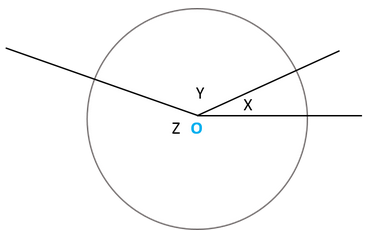In the given figure, three angles X, Y, and Z are formed at the centre O of the circle. If the angles X, Y, and Z are in the ratio 1:3:5 respectively, then find the value of Y.

Solution:

Step 1: Question statement and Inferences

We are given a circle with centre O. Three lines originate from the point O and form three angles X, Y, and Z at the centre.

These angles are in the ratio 1:3:5 i.e.

⇒ X : Y : Z = 1 : 3 : 5

We have to find the value of Y.

Step 2: Finding required values

Let’s say:

X = p

This means,

Y = 3p

Z = 5p

Now, we know that the sum of all the angles formed around a point is 360o. So,

X + Y + Z = 360o

p + 3p + 5p = 360o

p = 40o

Step 3: Calculating the final answer

So, the measure of the angle Y is 3p = 3*40o =120o

QUESTION: 8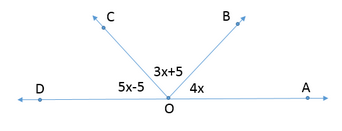In the figure above, AOD is a straight line. Find the measure of ∠AOB

Solution:

Step 1: Question statement and Inferences

We are given a straight line AOD.  ∠AOB, ∠BOC and ∠COD

, are the angles formed on the same side of the straight line. Also, the values of the angles are as follows:

∠AOB = 4x

∠BOC  = 3x +5

∠COD =  5x -5

Now, we have to find the value of  ∠AOB

. For that, first we need to find the value of x.

Step 2: Finding required values

Now, we know that the sum of all the angles formed on a straight line is equal to 180o.

Thus,

∠AOB + ∠BOC+ ∠COD = 1800

4x +3x+5+5x -5 = 1800

12x =1800

Thus , x =15o

So,

∠AOB = 4 x 15o = 60o

Step 3: Calculating the final answer

So, the measure of the angle AOB is 60 degrees.

QUESTION: 9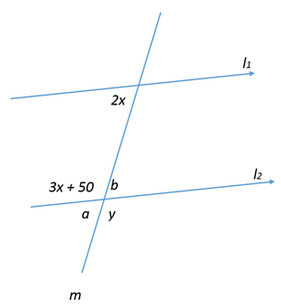In the given figure, if the lines l1 and l2 are parallel to each other, and the transversal line m intersects it. What is the value of y?

Solution:

Step 1: Question statement and Inferences

We are given that two parallel lines l1  and l2  are intersected by a transversal line m. Also, the angles formed are shown as 2x, 3x + 50, a, b, and y.

We have to find the value of angle y.

Now, to find the value of y, we need to establish the relation between x and y. Since the lines l1 and l2 are parallel lines and the transversal line m intersects them,

2x = a       (Corresponding angles)

Also, since

a = b   (Vertically opposite angles)

Hence,

b = 2x

Also,

(3x + 50) + a = 180º

(3x + 50) + 2x = 180º

5x + 50 = 180º

5x = 130º

x = 26º         ……………………… (1)

Step 2: Finding required values

Now, we know that the sum of all the angles formed around a point is 360o . So,

a + b + (3x + 50) + y = 360º

Thus,

2x + 2x + y + y = 360º

7x + y + 50 = 360º

7x + y = 310º    …… (2)

By putting the value of x from equation (1) to equation (2), we get:

7*26 + y = 310º

182   + y = 310º

y = 128º

Step 3: Calculating the final answer

So, the value of y = 128º

QUESTION: 10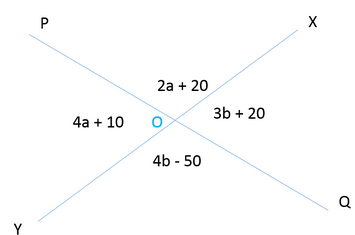In the given figure, what is the value of a + b, if both a and b are positive integers?

Solution:

Step 1: Question statement and Inferences

We are given that two lines XY and PQ intersect each other at point O. The measures of the four angles thus formed are given in terms of the positive integers a and b. These angles are:

∠POX=2a+20

∠XOQ=3b+20

∠QOY=4b–50

∠YOP=4a+10

We need to find the values of a and b to get the value of “a + b”.

Now, we know that the sum of all the angles formed around a point is 360. So,

∠POX+∠XOQ+∠QOY+∠YOP=360∘

By putting the values of these angles in the above equation, we get:

(2a + 20) + (3b + 20) + (4b – 50) + (4a + 10) = 360o

6a + 7b = 360o     …………….. (1)

Also, we know that the vertically opposite angles formed between straight lines are equal, so:

∠POX=∠YOQ

2a + 20 = 4b – 50

2a = 4b – 70

a = 2b – 35       ……………… (2)

And,

∠POY=∠XOQ

4a + 10 = 3b + 20

4a = 3b + 10          …………………. (3)

Step 2: Finding required values

By putting the value of a from equation (2) in equation (1),

6a + 7b = 360o

6(2b – 35) + 7b = 360o

12b – 210 + 7b = 360o

19b = 570o

b = 30o

Now, let’s plug the value of b in equation (2):

a = 2b – 35

a = 2(30o) – 35

a = 25o

Thus,

a + b = 30+ 25o

a + b = 55o

Step 3: Calculating the final answer

So, the sum of the values of a and b is 55.

QUESTION: 11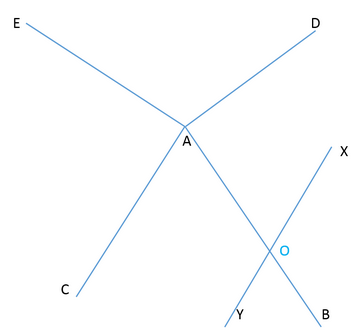In the figure above, AD bisects angle BAE and AE bisects angle CAD. Also, the line XY and AC are parallel to each other. Find the value of ∠DAE

.

(1)  ∠BAC = 60o

(2) ∠XOB = 120

Solution:

Steps 1 & 2: Understand Question and Draw Inferences

We are given that in the figure line AD bisects   and the line AE bisects angle  . We have to find the value of the  .

Now, from equation (1), (2), and (3)

∠DAE + ∠DAE + ∠DAE + ∠BAC=360

⇒3∠DAE+∠BAC=360∘.......(4) Also, since AC and XY are parallel to each other, ∠BAC =∠BOY (Corresponding angles).......(5)

However,wedon′tknowthevalueof∠BOY.

So,tofindthevalueof∠DAE,weneedtofindthevalueofeither∠BACor∠BOY.

Step 3: Analyse Statement 1

Statement 1 says: ∠BAC= 60o

This statement directly gives us the value of ∠BAC. By putting this value in equation (4), we get:

3∠DAE+∠BAC=360∘3∠DAE+60=360∘3∠DAE=300∘⇒∠DAE=100∘

Hence, statement (2) alone is sufficient to answer the question: What is the value of ∠BAE?

Step 4: Analyse Statement 2

Statement 2 says: ∠XOB= 120

Now, we know that the sum of all the angles formed around a point is 360. So,

∠AOX+∠XOB+∠BOY+∠YOA=360∘.......(6)Also,∠AOX=∠BOY∠XOB=∠YOA(Verticallyoppositeangles)Thus,equation(6)becomes:2∠XOB+2∠BOY=360∘2×120∘+2∠BOY=360∘2∠BOY=120∘∠BOY=60∘Fromequation(5),∠BAC=∠BOYThus,∠BAC=60∘Byputtingthisvalueinequation(4),weget:3∠DAE+∠BAC=360∘3∠DAE+60=360∘3∠DAE=300∘⇒∠DAE=100∘

Hence, statement (2) alone is sufficient to answer the question: What is the value of ∠BAE?

Step 5: Analyse Both Statements Together (if needed)

Since statement (1) and (2) alone are sufficient to answer the question, we don’t need to perform this step.

Correct Choice : D

QUESTION: 12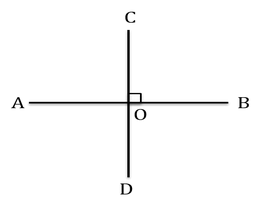Is the segment CD a perpendicular bisector of the segment AB?

(1) Half of CD = CO

(2) AO = DO

Solution:

Steps 1 & 2: Understand Question and Draw Inferences

We are given that segments AB & CD are perpendicular to each other, and we have to find: Does egment CD bisect segment AB? (The perpendicular part is omitted as we already know from the square symbol used at the point O that the segments are perpendicular to each other.)

So, the question becomes, is AO = OB?

Since we are not given any other information, let’s move on to the analysis of the statements.

Step 3: Analyse Statement 1

Statement I says: Half of CD = CO

It means that point O is the mid-point of CD or we can say that segment AB bisects segment CD.

Did we get the answer to the question? Well, no!

Pay attention to the question, Is segment CD, a perpendicular bi-sector of segment AB? It is not answered. We seek for an answer whether CD bi-sects AB and not vice-versa. Had you hurriedly done this, you would have been trapped laid by the test-maker!

Take Away: Pay attention to the verbiage!

Hence, statement I is not sufficient to answer the question: is AO = OB?

Step 4: Analyse Statement 2

Statement II says: AO = DO

It does not help in any way. It simply builds a relationship between sub-parts of two different segments. We are interested in whether AO = OB. This question is still not answered.

Hence, statement II is not sufficient to answer the question: is AO = OB?

Step 5: Analyse Both Statements Together (if needed)

Since step 3 and step 4 were not able to provide the answer to the question, let’s analyse both the statements together:

Statement I:

Segment AB bisects segment CD.

Statement II:

AO = DO.

Even the combination of both the statements does not help us to find the answer to our question.

Hence, both statements combined together are not sufficient to answer the question.

QUESTION: 13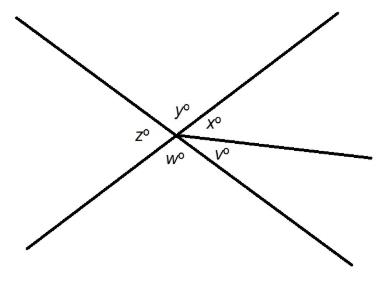Refer to the above diagram. Evaluate w.

Statement 1: x=2v

Statement 2: y=2x

Solution:

Statement 1 alone gives insufficient information. It only gives a relationship between x and v, but no further clues about the measures of any angle are given.

Statement 2 alone gives insufficient information; w=y=2x, since the angles with those measures are vertical; since no measures are known, w cannot be calculated.

Now assume both statements are true. Again, from Statement 1, x=2v; from Statement 2, y=2x=2(2v)=4v. Again, from the diagram, w=y=4v. Three angles with measures x,v,w together form a straight angle, so

x+v+w=180

2v+v+4v=180

7v=180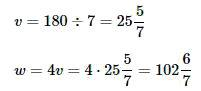Therefore, both statements together are sufficient to answer the question.

QUESTION: 14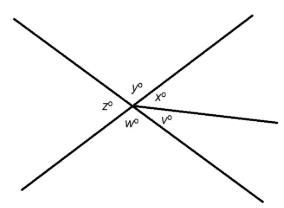Refer to the above diagram. Evaluate v.

Statement 1: x+y=150

Statement 2: w+v=130

Solution:

Assume Statement 1 alone. From the diagram, the three angles of measure x,y,v together form a straight angle, so
x+y+v=180
From Statement 1,
x+y=150,
so by the subtraction property of equality,
x+y+v−(x+y)=180−150
v=30
Assume Statement 2 alone. w+v=130, but there is no clue about the value of w or any other angle measure, so the value of v cannot be computed.

QUESTION: 15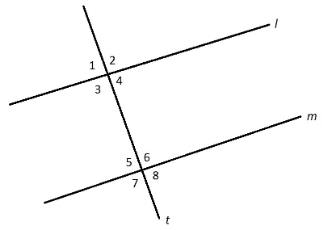Evaluate m∠1.
Statement 1: m∠4=88∘
Statement 2: m∠8=88∘

Solution:

Assume Statement 1 alone. ∠1 and ∠4 are vertical angles, so they must have the same measure; m∠1=m∠4=88∘.

Assume Statement 2 alone. ∠1 and ∠8 are alternating exterior angles, which are congruent if and only if l||m; however, we do not know whether l||m, so no conclusions can be made about m∠1.Use Code STAYHOME200 and get INR 200 additional OFF Use Coupon Code

Track your progress, build streaks, highlight & save important lessons and more!

Similar Content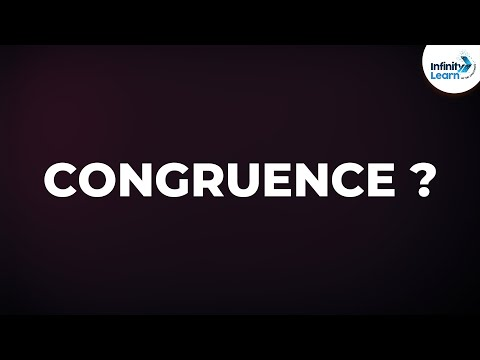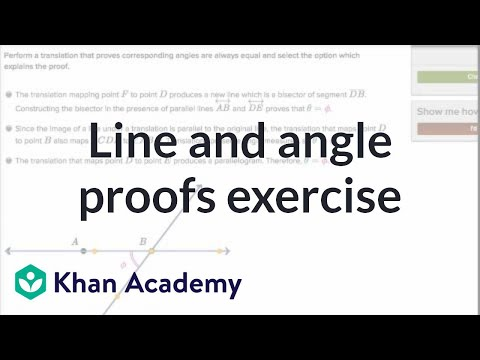Related tests